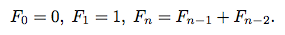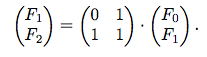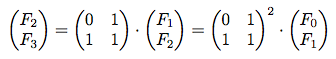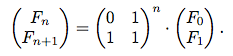On the first day of my Algorithms class here at school, we learned three ways to implement the well known Fibonacci series generating algorithm.

## Standard recursive

Everyone and their mom knows the recursive way to implement Fibonacci:

``````def fib(n)
return 1 if n < 2
fib(n-1) + fib(n-2)
end
``````

This is an extremely inefficient algorithm - the running time is exponential, growing almost as fast as the Fibonacci numbers themselves! For example, to find the 200th Fibonacci number, the algorithm takes 2138 steps! Obviously this is not feasible to compute even on a modern computer.

What's the problem with this algorithm? We can see that we are repeatedly computing the value of each Fibonacci number (ex. for the 4th fibonacci number, we need to add the 3rd and 2nd, and to calculate the 3rd, we need to add the 2nd and 1st, and etc).

## Memoization

One way we can avoid this double-counting is to remember what Fibonacci values were already computed in a hash table so we don't have to launch into another recursive call for a value we already have. We can also switch to implementing this with an iterative method:

``````def fib(n)
fibHash = [1, 1]
for i in 2..n
fibHash.append(fibHash[i-1]+fibHash[i-2]
return fibHash[n]
end
``````

Now this is pretty clever, but who knew that there was an even MORE clever mathematical way to tackle this problem?

Consider the following equations that represent the Fibonacci series:We can write the first two equations in matrix notation:The rest of the equations may be written in matrix notation, and if we substitute the value for [F1 F2] from the first equation, we notice a pattern:Now wait a minute - have we really figured out a way to make an O(1) algorithm for the Fibonacci series?

Unfortunately, we need to take the multiplication and power operation runtimes into account because they are computationally more expensive than doing addition on large numbers. With the performance cost of using multiplication, we see that that runtime of this matrix implementation is actually O(n2), which is sadly not faster than our O(n) algorithm.

Credit: Material and screenshots taken from Algorithms by S. Dasgupta, C.H. Papadimitriou, and U.V. Vazirani.